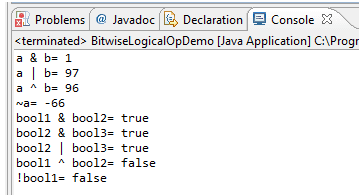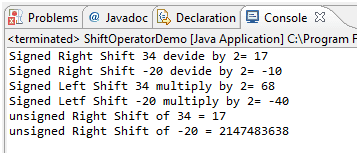# Java Logical Operators

## Description

Sometimes, whether a statement is executed is determined by a combination of several conditions.You can use logical operators to combine these conditions. Logical operators are known as Boolean operators or bitwise logical operators. The boolean operator operates on boolean values to create a new boolean value. The bitwise logical operators are “&”, “|”, “^”, and “~” or “!”. The following table shows the outcome of each operation.

a b a&b a|b a^b ~a or !a
true(1) true(1) true true false false
true(1) false(0) false true true false
false(0) true(1) false true true true
false(0) false(0) false false false true

The NOT Operator

Also called the bitwise complement, the unary NOT operator, ~, inverts all of the bits of its operand. If applied on integer operand it will reverse all bits similarly if applied to boolean literal it will reverse it.

```int a =23; // 23 is represented in binary as 10111
int b = ~a; // this will reverts the bits 01000 which is 8 in decimal
boolean x = true;
boolean y = !x;  // This will assign false value to y as x is true
```

The AND Operator

The AND operator “&” produces a 1 bit if both operands are 1 otherwise 0 bit. Similarly, for boolean operands, it will result in true if both operands are true else result will be false.

```int var1 = 23; //boolean value would be 010111
int var2 = 33; //boolean value would be 100001
int var3=var1 & var2 // result in binary 000001 & in decimal 1
boolean b1 = true;
boolean b2=false;
boolean b3 = b1&b2; // b3 would be false
```

The OR Operator

The OR operator “|” produces a 0 bit if both operands are 0 otherwise 1 bit. Similarly, for boolean operands, it will result in false if both operands are false else result will be true.

```int var1 = 23; //boolean value would be 010111
int var2 = 33; //boolean value would be 100001
int var3=var1 | var2 // result in binary 110111& in decimal 55
boolean b1 = true;
boolean b2=false;
booleanb3 = b1|b2; // b3 would be true
```

The XOR (exclusive OR) Operator

The XOR operator “^” produces a 0 bit if both operands are same (either both 0 or both 1) otherwise 1 bit. Similarly, for boolean operands, it will result in false if both operands are same (either both are false or both true) else result will be true.

```iint var1 = 23; //boolean value would be 010111
int var2 = 33; //boolean value would be 100001
int var3=var1 ^ var2 // result in binary 110110& in decimal 54
boolean b1 = true;
boolean b2=false;
booleanb3 = b1 ^ b2; // b3 would be true
```

Let’s look at below program which demonstrate above operators for boolean and integer operation.

Java Code: Go to the editor

``````public class BitwiseLogicalOpDemo {
public static void main(String[] args) {
//Integer bitwise logical operator
int a = 65; // binary representation 1000001
int b = 33; // binary representation 0100001
System.out.println("a & b= " + (a & b));
System.out.println("a | b= " + (a | b));
System.out.println("a ^ b= " + (a ^ b));
System.out.println("~a= " + ~a);
//boolean logical operator
boolean bool1 = true;
boolean bool2 = true;
boolean bool3 = false;
System.out.println("bool1 & bool2= " + (bool1 & bool2));
System.out.println("bool2 & bool3= " + (bool2 | bool3));
System.out.println("bool2 | bool3= " + (bool2 | bool3));
System.out.println("bool1 ^ bool2= " + (bool1 ^ bool2));
System.out.println("!bool1= " + !bool1);
}

}
```
```

Output:Bitwise Shift Operators:

>> (Signed right shift):

In Java, the operator “>>” is signed right shift operator. All integers are signed in Java, and it is fine to use >> for negative numbers. The operator “>>” uses the sign bit (left most bit) to fill the trailing positions after shift. If the number is negative, then 1 is used as a filler and if the number is positive, then 0 is used as a filler. In simple terms it will divide the number by 2 to power number of shifted bit

```int a =8; //binary representation is 1000
System.out.println(a>>1); //This will print 4 (binary representation 0100)
```

<< (Singed left shift):

This operator moves all bits to the left side (simply multiply the number by two to power number of bits shifted).

```int a =8; //binary representation is 1000
System.out.println(a<<2); //This will print 32 (binary representation 100000)
```

>>> (Unsigned right shift) :

As you have just seen, the >> operator automatically fills the high-order bit with its previous contents each time a shift occurs. This preserves the sign of the value. However, sometimes this is undesirable. For example, if you are shifting something that does not represent a numeric value, you may not want sign extension to take place. First bit represents a sign of the integer.

```int a =-2; // This is represented in binary as 10000000 0000000000000000 000000010
System.out.println(a>>>1);
//01000000 00000000 00000000 00000001 in decimal=2147483647
```

Below program demonstrate shift operators.

Java Code: Go to the editor

``````public class ShiftOperatorDemo {
public static void main(String[] args) {
int a = 34;  //binary representation 00000000 00000000 00000000 00100010
int b = -20; //binary representation 10000000 00000000 00000000 00010100
System.out.println("Signed Right Shift 34 devide by 2= " + (a>>1) );
System.out.println("Signed Right Shift -20 devide by 2= " + (b>>1) );
System.out.println("Signed Left Shift 34 multiply by 2= " + (a<<1) );
System.out.println("Signed Letf Shift -20 multiply by 2= " + (b<<1) );
System.out.println("unsigned Right Shift of 34 = " + (a>>>1) );
System.out.println("unsigned Right Shift of -20 = " + (b>>>1) );
}
}
```
```

Output:Summary

• Logical operators are known as Boolean operators or bitwise logical operators.
• Logical operators are & (and), | (or), ^ (ex-or), !/~ (not).
• Binary Shift operators are >> (right shift), << (left shift), >>> (unsigned right shift).

Java Code Editor:

Previous: Conditional Operator
Next: Switch Satement

﻿